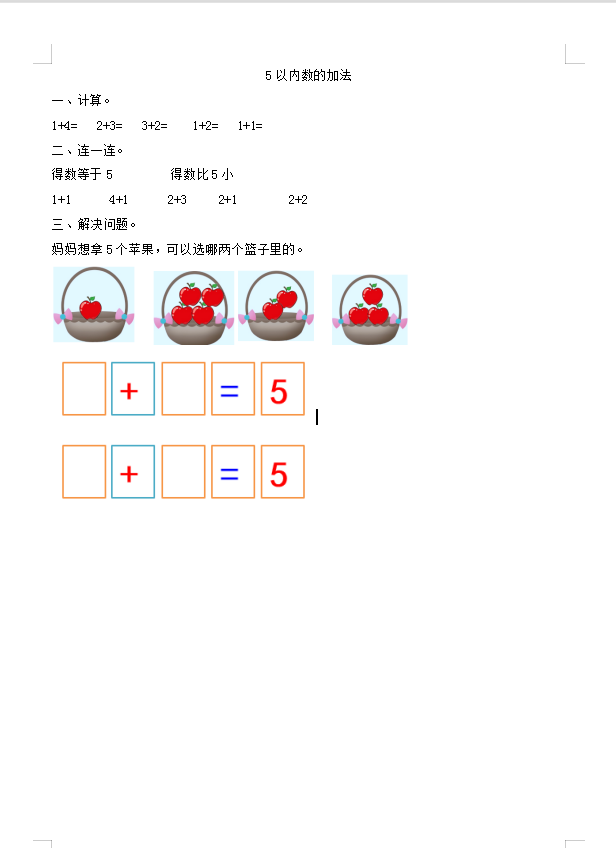5以内数的加法

1+4=   2+3=   3+2=    1+2=   1+1=

1+1      4+1      2+3     2+1        2+2